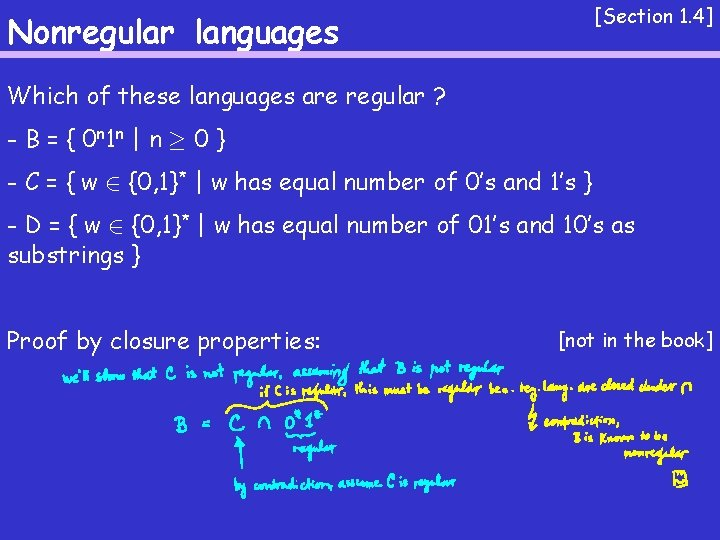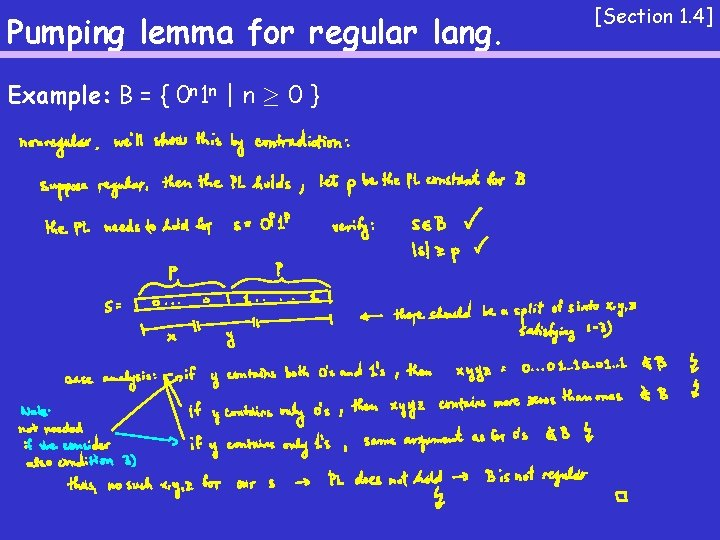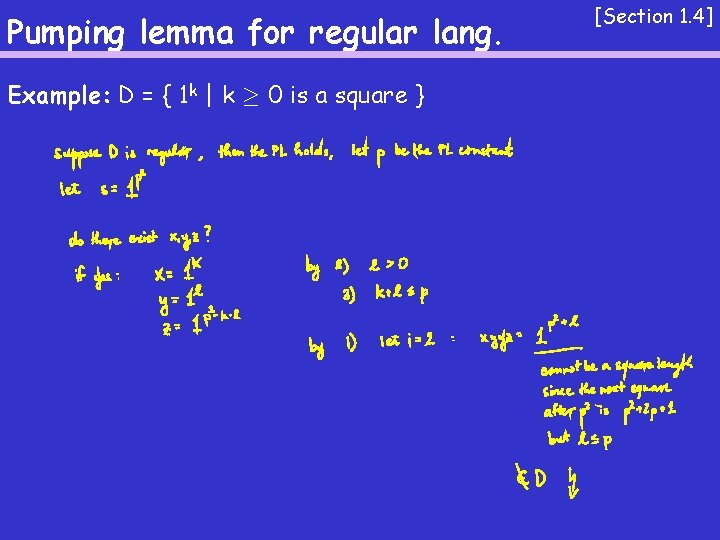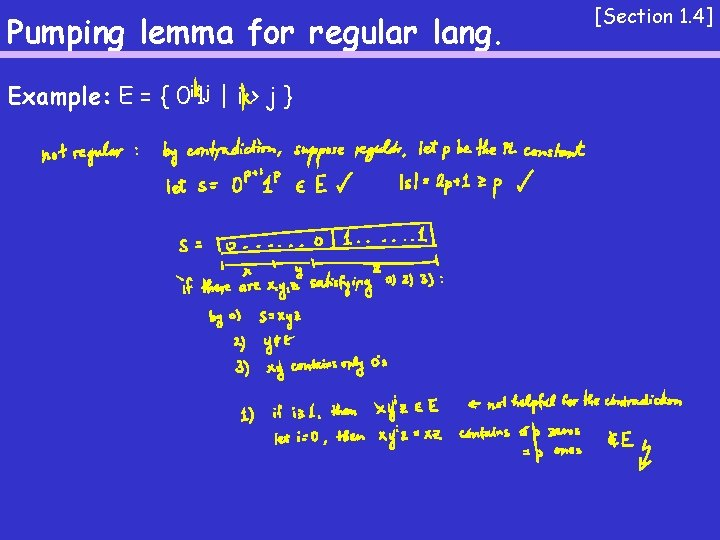# Nonregular languages Section 1 4 Which of these

• Slides: 9
Download presentationNonregular languages [Section 1. 4] Which of these languages are regular ? - B = { 0 n 1 n | n ¸ 0 } - C = { w 2 {0, 1}* | w has equal number of 0’s and 1’s } - D = { w 2 {0, 1}* | w has equal number of 01’s and 10’s as substrings }[Section 1. 4] Nonregular languages Which of these languages are regular ? - B = { 0 n 1 n | n ¸ 0 } - C = { w 2 {0, 1}* | w has equal number of 0’s and 1’s } - D = { w 2 {0, 1}* | w has equal number of 01’s and 10’s as substrings } Proof by closure properties: [not in the book]Pumping lemma for regular lang. [Section 1. 4] Suppose we have a DFA with p states. Suppose there is a string of length > p that is accepted. Are there other strings that are accepted?Pumping lemma for regular lang. [Section 1. 4] Thm 1. 70[pumping lemma]: Let A be a regular language. Then there exists a number p s. t. for every string s 2 A of length ¸ p there exist strings x, y, and z s. t. 0. s = xyz, 1. For each i ¸ 0, xyiz 2 A, 2. |y| > 0, and 3. |xy| · p.Pumping lemma for regular lang. Example: B = { 0 n 1 n | n ¸ 0 } [Section 1. 4]Pumping lemma for regular lang. [Section 1. 4] Example: C = { w | w has equal number of 0’s and 1’s }Pumping lemma for regular lang. Example: F = { ww | w 2 {0, 1}* } [Section 1. 4]Pumping lemma for regular lang. Example: D = { 1 k | k ¸ 0 is a square } [Section 1. 4]Pumping lemma for regular lang. Example: E = { 0 i 1 j | i > j } [Section 1. 4]